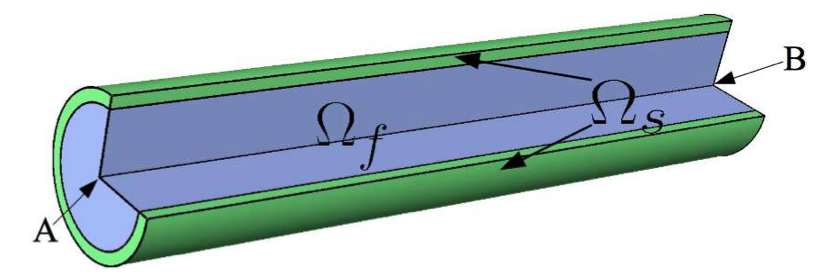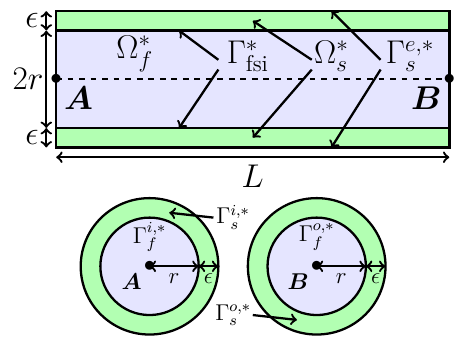# Wave Pressure 3D

Computer codes, used for the acquisition of results, are from Vincent [Chabannes].

## 1. Problem Description

As in the 2D case, the blood flow modelisation, by observing a pressure wave progression into a vessel, is the subjet of this benchmark. But this time, instead of a two-dimensional model, we use a three-dimensional model, with a cylinderFigure 1 : Geometry of three-dimensional elastic tube.

This represents the domains into the initial condition, with $\Omega_f$ and $\Omega_s$ respectively the fluid and the solid domain. The cylinder radius equals to $r+\epsilon$, where $r$ is the radius of the fluid domain and $\epsilon$ the part of the solid domain.Figure 2 : Sections of the three-dimensional elastic tube.

$\Gamma^*_{fsi}$ is the interface between the fluid and solid domains, whereas $\Gamma^{e,*}_s$ is the interface between the solid domain and the exterior. $\Gamma_f^{i,*}$ and $\Gamma_f^{o,*}$ are respectively the inflow and the outflow of the fluid domain. Likewise, $\Gamma_s^{i,*}$ and $\Gamma_s^{o,*}$ are the extremities of the solid domain.

During this benchmark, we will study two different cases, named BC-1 and BC-2, that differ from boundary conditions. BC-2 are conditions imposed to be more physiological than the ones from BC-1. So we waiting for more realistics based results from BC-2.

### 1.1. Boundary conditions

• on $\Gamma_f^{i,*}$ the pressure wave pulse

\boldsymbol{\sigma}_{f} \boldsymbol{n}_f = \left\{ \begin{aligned} & \left(-\frac{1.3332 \cdot 10^4}{2} \left( 1 - \cos \left( \frac{ \pi t} {1.5 \cdot 10^{-3}} \right) \right), 0 \right)^T \quad & \text{ if } t < 0.003 \\ & \boldsymbol{0} \quad & \text{ else } \end{aligned} \right.
$\frac{\partial \boldsymbol{\eta_{s}} }{\partial t} - \boldsymbol{u}_f \circ \mathcal{A}_{f}^t = \boldsymbol{0} , \quad \text{ on } \Gamma_{fsi}^* \times \left[t_i,t_f \right] \quad \boldsymbol{(1)}$
$\boldsymbol{F}_{s} \boldsymbol{\Sigma}_{s} \boldsymbol{n}^*_s + J_{\mathcal{A}_{f}^t} \hat{\boldsymbol{\sigma}}_f \boldsymbol{F}_{\mathcal{A}_{f}^t}^{-T} \boldsymbol{n}^*_f = \boldsymbol{0} , \quad \text{ on } \Gamma_{fsi}^* \times \left[t_i,t_f \right] \quad \boldsymbol{(2)}$
$\boldsymbol{\varphi}_s^t - \mathcal{A}_{f}^t = \boldsymbol{0} , \quad \text{ on } \Gamma_{fsi}^* \times \left[t_i,t_f \right] \quad \boldsymbol{(3)}$

Then we have two different cases :

• Case BC-1

• on $\Gamma_f^{o,*}$ : $\boldsymbol{\sigma}_{f} \boldsymbol{n}_f =0$

• on $\Gamma_s^{i,*} \cup \Gamma_s^{o,*}$ a null displacement : $\boldsymbol{\eta}_s=0$

• on $\Gamma^{e,*}_{s}$ : $\boldsymbol{F}_s\boldsymbol{\Sigma}_s\boldsymbol{n}_s^*=0$

• on $\Gamma_f^{i,*}U \Gamma_f^{o,*}$ : $\mathcal{A}^t_f=\boldsymbol{\mathrm{x}}^*$

• Case BC-2

• on $\Gamma_f^{o,*}$ : $\boldsymbol{\sigma}_{f} \boldsymbol{n}_f = -P_0\boldsymbol{n}_f$

• on $\Gamma_s^{i,*}$ a null displacement $\boldsymbol{\eta}_s=0$

• on $\Gamma^{e,*}_{s}$ : $\boldsymbol{F}_s\boldsymbol{\Sigma}_s\boldsymbol{n}_s^* + \alpha \boldsymbol{\eta}_s=0$

• on $\Gamma^{o,*}_{s}$ : $\boldsymbol{F}_s\boldsymbol{\Sigma}_s\boldsymbol{n}_s^* =0$

• on $\Gamma_f^{i,*}$ : $\mathcal{A}^t_f=\boldsymbol{\mathrm{x}}^*$

• on $\Gamma_f^{o,*}$ : $\nabla \mathcal{A}^t_f \boldsymbol{n}_f^*=\boldsymbol{n}_f^*$

### 1.2. Initial conditions

The chosen time step is $\Delta t=0.0001$

## 2. Inputs

Table 1. Fixed and Variable Input Parameters
Name Description Nominal Value Units

$E_s$

Young’s modulus

$3 \times 10^6$

$dynes.cm^{-2}$

$\nu_s$

Poisson’s ratio

$0.3$

dimensionless

$r$

0.5

$cm$

$\epsilon$

0.1

$cm$

$L$

tube length

5

$cm$

$A$

A coordinates

(0,0,0)

$cm$

$B$

B coordinates

(5,0,0)

$cm$

$\mu_f$

viscosity

$0.03$

$poise$

$\rho_f$

density

$1$

$g.cm^{-3}$

$R_p$

proximal resistance

$400$

$R_d$

distal resistance

$6.2 \times 10^3$

$C_d$

capacitance

$2.72 \times 10^{-4}$

## 3. Outputs

After solving the fluid struture model, we obtain $(\mathcal{A}^t, \boldsymbol{u}_f, p_f, \boldsymbol{\eta}_s)$

with $\mathcal{A}^t$ the ALE map, $\boldsymbol{u}_f$ the fluid velocity, $p_f$ the fluid pressure and $\boldsymbol\eta_s$ the structure displacement.

## 4. Discretization

Here are the different configurations we worked on. The parameter `Incomp` defines if we use the incompressibility constraint or not.

 Config Fluid Structure $N_{elt}$ $N_{geo}$ $N_{dof}$ $N_{elt}$ $N_{geo}$ $N_{dof}$ Incomp $(1)$ $13625$ $1~(P2P1)$ $69836$ $12961$ $1$ $12876~(P1)$ No $(2)$ $13625$ $1~(P2P1)$ $69836$ $12961$ $1$ $81536~(P1)$ Yes $(3)$ $1609$ $2~(P3P2)$ $30744$ $3361$ $2$ $19878~(P2)$ No

For the structure time discretization, we will use Newmark-beta method, with parameters $\gamma=0.5$ and $\beta=0.25$.

And for the fluid time discretization, BDF, at order $2$, is the method we choose.

These two methods can be found in [Chabannes] papers.

## 5. Results

 Documentation pending

### 5.1. Conclusion

 Documentation pending

## 6. Bibliography

References for this benchmark
• [Chabannes] Vincent Chabannes, Vers la simulation numérique des écoulements sanguins, Équations aux dérivées partielles [math.AP], Université de Grenoble, 2013.